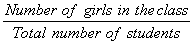## Definition Of Fraction Notation

If two numbers are written in the form of a/b , then they are said to be
in Fraction Notation, where 'a' ≠ 0 and 'b' ≠ 0.

In a fraction, the number written above the bar is called "numerator" and the one written below the bar is called "denominator".

### Example of Fraction NotationIn the given figure, 5 out of 8 flowers are shaded.
To write the fraction notation of the shaded part, we first note the numerator and denominator.
Total number of parts will be written as the denominator.
Number of parts that are shaded will be the numerator.
Here, 5 out of 8 flowers have been shaded.
So, 5 is the numerator and 8 is the denominator.
So, we write the fraction as 5/8.

### Solved Example on Fraction Notation

#### There are 18 boys and 19 girls in a class of 37 students. What fraction of the class are the girls?

##### Choices:

A.19/37
B. 1/37
C. 1/18
D. 18/37

Step 4: Fraction of the class that is girls == 19/37 [Substitute the values.]